Lec 25 - Inverse

Wednesday, March 07, 2012

9:29 AM

Recall: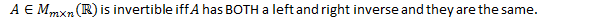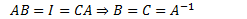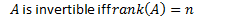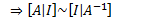Eg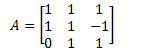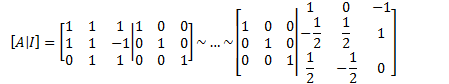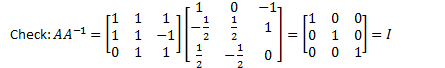Eg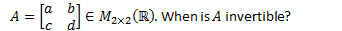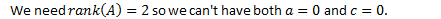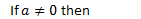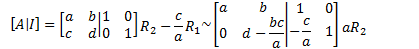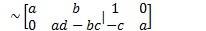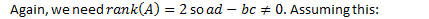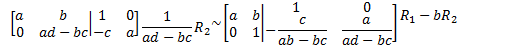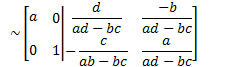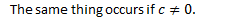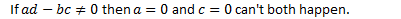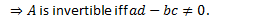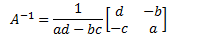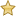Theorem: Invertible Matrix Theorem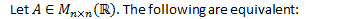1.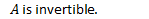1.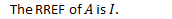2.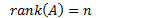3.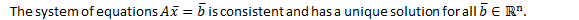4.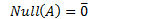5.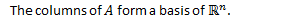6.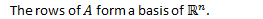7.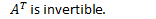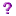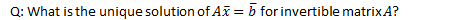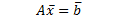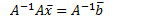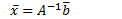Eg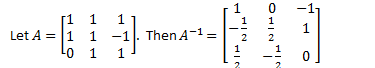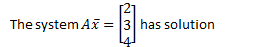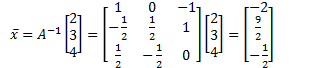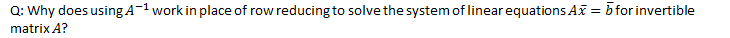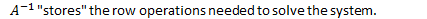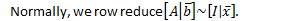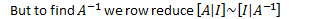The row operations to get both RREF matrices are the same.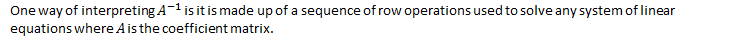Elementary Matrices

Definition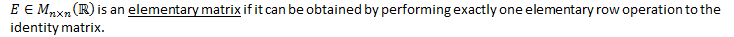Eg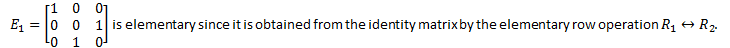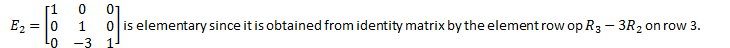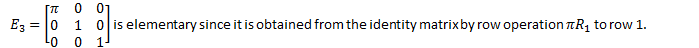Theorem

Every elementary matrix is invertible.

Proof:

For every elementary row operation, there is an inverse element row operation to undo it.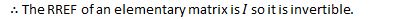Q.E.D.

In fact, the inverse of any elementary matrix is the element matrix representing the inverse elementary row operation.

Eg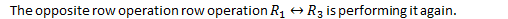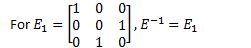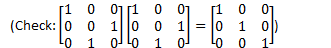Eg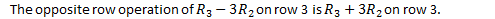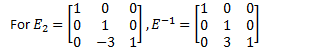Eg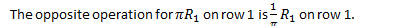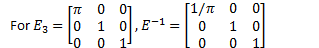Eg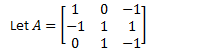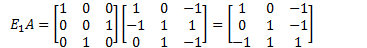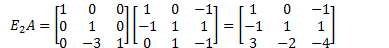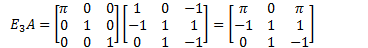Created by Tim Pei with Microsoft OneNote 2010
One place for all your notes and information